Checkout JEE MAINS 2022 Question Paper Analysis : Checkout JEE MAINS 2022 Question Paper Analysis :

# JEE Main 2021 March 16 – Shift 1 Maths Question Paper with Solutions

JEE Main 2021 question paper for Maths (March 16 – Shift 1) is provided here along with solutions that have been exclusively created by our subject experts. Candidates will find the Maths solved questions and these will help them to check the correct answers as well as analyze their performance. Students can download the JEE Main 2021 March 16th Shift 1 Maths Question Paper in a PDF format for studying and practicing offline to improve their speed and accuracy.

### JEE Main 2021 March 16th Shift 1 Maths Question Paper

Section A

Question 1: Consider three observations a, b and c such that b = a+c. If the standard deviation of a+2, b+2, c+2 is d, then which of the following is true?

1. a. b2 = a2 + c2 + 3d2
2. b. b2 = 3 (a2 + c2) - 9d2
3. c. b2 = 3 (a2 + c2) + 9d2
4. d. b2 = 3 (a2 + c2 + d2)

Solution:

For a, b, c mean =

$$\bar{x}$$
= [a + b + c] / 3

$$\bar{x}$$
= 2b / 3

S.D. of a, b, c = dd2 = [a2 + b2 + c2] / 3 - (4b2 / 9)b2 = 3a2 + 3c2 – 9d2

Question 2: Let a vector

$$\alpha \hat{i}+\beta \hat{j}$$
be obtained by rotating the vector
$$\sqrt{3} \hat{i}+\hat{j}$$
by an angle 45° about the origin in counterclockwise direction in the first quadrant. Then the area of triangle having vertices (ɑ, β), (0, β) and (0, 0) is equal to:

1. a. 1
2. b. 1 / 2
3. c. 1 / √2
4. d. 2 √2

Solution: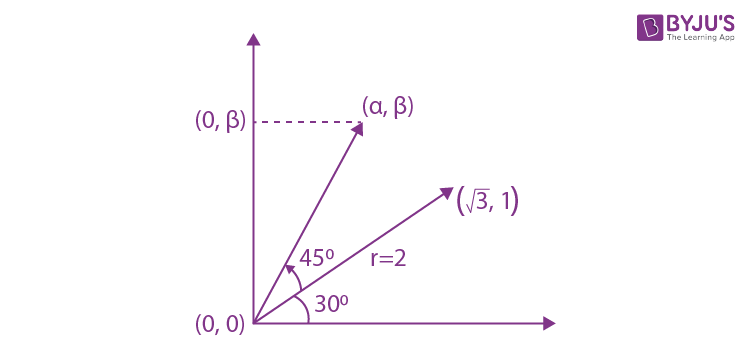(ɑ, β) = (2 cos 75o, 2 sin 75o)

Area = (1 / 2) (2 cos 75°)(2 sin 75°)

= sin (150°)

= 1 / 2 square unit

Question 3: If for a>0, the feet of perpendiculars from the points A (a, –2a, 3) and B (0, 4, 5) on the plane lx + my + nz = 0 are points C (0, –a, –1) and D respectively, then the length of line segment CD is equal to :

1. a. √41
2. b. √55
3. c. √31
4. d. √66

Solution: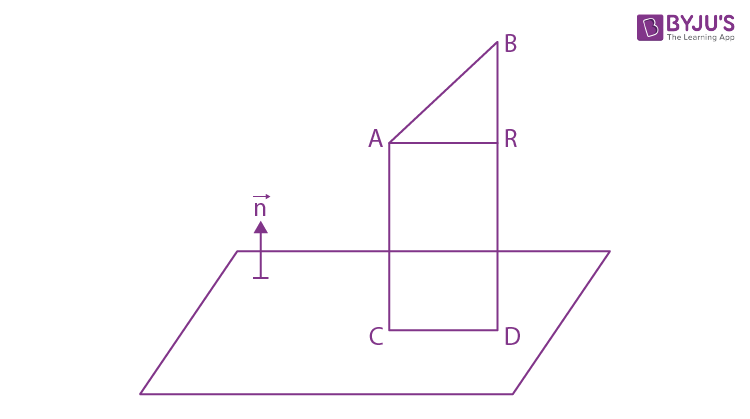Direction cosines of plane = λ (direction cosines of line AC)

Direction cosines of plane = λa, - λa, 4λ

Hence equation plane is: ax - ay + 4z = 0

The point C lies on the plane

a (0) − a (− a)+ 4 (−1) = 0 ⇒ a = 2 (∵ a > 0)

So plane is 2x − 2y + 4z = 0, C ≡ (0,−2,−1)

So for coordinates of D,

[x - 0] / 2 = [y - 4] / - 2 = [z - 5] / 4 = - {[2 (0) - 2 (4) + 4 (5)] / [22 + 22 + 42]}

D ≡ (- 1, 5, 3)

CD = √66 units

Question 4: The range of a ∈ R for which the function f (x) = (4a – 3) (x + loge 5) + 2 (a – 7) cot (x / 2) sin2 (x / 2), x ≠ 2nπ, n ∈ N has critical points, is

1. a. [- 4 / 3, 2]
2. b. [1, ∞)
3. c. (- ∞, - 1]
4. d. (– 3, 1)

Solution:

f (x) = (4a – 3) (x + ln 5) + 2(a – 7) [(cos (x / 2) / sin (x / 2)) * sin2 (x / 2)]

f (x) = (4a – 3) (x + loge5) + (a – 7) sin x

f’ (x) = (4a – 3) + (a – 7) cos x = 0

cos x = - (4a - 3) / (a - 7)

- 1 ≤ - (4a - 3) / (a - 7) ≤ 1 (because - 1 ≤ cos x ≤ 1)

- 1 < [4a - 3] / [a - 7] ≤ 1

{[4a - 3] / [a - 7]} - 1 ≤ 0 and {[4a - 3] / [a - 7]} + 1 > 0

a.∈ [4 / 3, 7) and a ∈ (- ∞, 2) ⋃ (7, ∞)

(- 4 / 3) ≤ a < 2

Question 5: Let the functions f: R→R and g: R →R be defined as:

$$f(x)=\left\{\begin{matrix} x+2, &x\leq 0 \\ x^2& x\geq 0 \end{matrix}\right.$$
and
$$g(x)=\left\{\begin{matrix} x^3, &x<1 \\ 3x-2& x\geq 1 \end{matrix}\right.$$
Then, the number of points in R where (fog)(x) is NOT differentiable is equal to:

1. a. 1
2. b. 2
3. c. 3
4. d. 0

Solution:

$$fog(x))=\left\{\begin{matrix} x^3+2, &x<0 & \\ x^6,&0\leq x< 1 & \\ (3x-2)^2,& x\geq 1 & \end{matrix}\right.$$

Clearly fog(x) is discontinuous at x = 0 then non-differentiable at x = 0

Now,

at x = 1

RHD = limh→0+ [f (1 + h) - f (1) ] / [h] = limh→0+ {[3 (1 + h) - 2]2 - 1} / h = 6

LHD = limh→0- [f (1 - h) - f (1) ] / [- h] = limh→0- [(1 - h)6 - 1] / - h = 6

Number of points of non-differentiability = 1

Question 6:Let a complex number z, |z| ≠ 1, satisfy log1/√2 [(|z| + 11) / (|z| - 1)2] ≤ 2. Then, the largest value of |z| is equal to ____

1. a. 5
2. b. 8
3. c. 6
4. d. 7

Solution:

[(|z| + 11) / (|z| - 1)2] ≥ 1 / 2

2|z| + 22 ≥ (|z| – 1)2

2|z| + 22 ≥ |z|2 – 2|z| + 1

|z|2 – 4|z| – 21 ≤ 0

(|z| – 7) (|z| + 3) ≤ 0

|z| ≤ 7

|z|max = 7

Question 7: A pack of cards has one card missing. Two cards are drawn randomly and are found to be spades. The probability that the missing card is not a spade is:

1. a. 3 / 4
2. b. 52 / 867
3. c. 39 / 50
4. d. 22 / 425

Solution:

$$P(\bar{S}_{missing}|both \ found \ spade)=\frac{P(\bar{S_m}\cap BFS)}{P(BFS)}$$

= [1 - (13 / 52) * (13 / 51) (12 / 50)] / [(1 - (13 / 52) * {(13 / 51) (12 / 50) + (13 / 52) (12 / 51) * (11 / 50)}]

= 39 / 50

Question 8: If n is the number of irrational terms in the expansion of [31/4 + 51/8]60, then (n - 1) is divisible by

1. a. 8
2. b. 26
3. c. 7
4. d. 30

Solution:

Tr+1 = 60Cr (31/4)60-r (51/8)r

It is rational if [60 - r] / 4, (r / 8), both are whole numbers, r ∈ {0,1,2,....60}

[60 - r] / 4 ∈ W ⇒ r ∈ {0, 4, 8, ……..60} and (r / 8) ∈ W ⇒ r ∈ {0,8,16,…56}

Common terms r ∈ {0,8,16,....56}

So 8 terms are rational

Then irrational terms = 61 – 8 = 53 = n

∴n – 1 = 52 = 13 × 22

The factors are 1,2,4,13,26,52.

Question 9: Let the position vectors of two points P and Q be 3i - j + 2k and i + 2j - 4k, respectively. Let R and S be two points such that the direction ratios of lines PR and QS are (4, –1, 2) and (–2,1,–2) respectively. Let lines PR and QS intersect at T. If the vector TA is perpendicular to both PR and QS and the length of vector TA is √5 units, then the modulus of a position vector of A is:

1. a. √5
2. b. √171
3. c. √227
4. d. √482

Solution:

P = 3i - j + 2k and Q = i + 2j - 4k

vPR = (4, - 1, 2) and vQS = (- 2 , 1, - 2)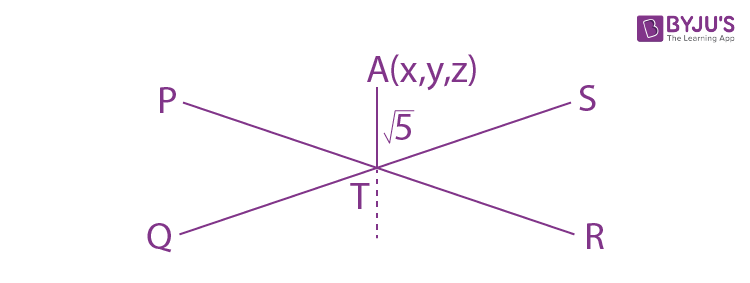LPR = (3i - j + 2k) + λ (4i - 1j + 2k)

LQS : r = (i + 2j - 4k) + 𝝁 (- 2i + 1j - 2k)

Now T on PR = (3 + 4λ, - 1 - λ, 2 + 2λ)

Similarly T on QS = (1 − 2𝝁, 2 + 𝝁,−4 − 2𝝁)

For λ and 𝝁:

3 + 4λ = 1 - 2𝝁 ⇒ 𝝁 + 2λ = - 1 ⇒ λ = 2

- 1 - λ = 2 + 𝝁 ⇒ 𝝁 + λ = - 3 ⇒ 𝝁 = - 5

And 2 + 2λ = - 4𝝁

T : (11, - 3, 6)

D.R. of TA = vQS x vPR

$$\begin{vmatrix} i &j &k \\ -2 &1 &-2 \\ 4 &-1 &2 \end{vmatrix}$$
= 0i - 4j - 2k

LTA : r = (11i - 3j + 6k) + λ (- 4j - 2k)

Now A = (11,− 3 − 4λ, 6 − 2λ)

TA = √5

(4λ)2 + (2λ)2 = 5

16λ2 + 4λ2 = 5

λ = ± 1 / 2

A: (11, –5, 5) or A: (11, –1, 7)

|A| = √(121 + 25 + 25) or |A| = √(121 + 1 + 49)

= √171 or √171

Question 10: If the three normals drawn to the parabola, y2 = 2x pass through the point (a, 0) a ≠ 0, then ‘a’ must be greater than

1. a. 1
2. b.1 / 2
3. c. - 1 / 2
4. d. –1

Solution:

Let the equation of the normal be y = mx – 2am – am3.

Here 4a = 2

a = 1 / 2

y = mx – m – (1 / 2) m3

It passes through A (a, 0) then

0 = am – m – (1 / 2) m3

m = 0, m2 - 2 (a - 1) = 0

For real values of m

2 (a – 1) > 0

a > 1

Question 11: Let Sk = ∑r=1k tan-1[(6r) / (2r+1 + 32r+1)]. Then limk→∞ Sk =

1. a. tan-1 (3 / 2)
2. b. cot-1 (3 / 2)
3. c. π / 2
4. d. tan–1(3)

Solution: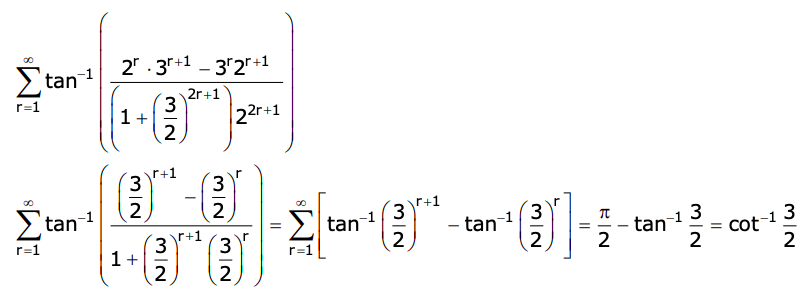Question 12: The number of roots of the equation, (81)sin2x + (81)cos2x = 30 in the interval [0, π] is equal to :

1. a. 3
2. b. 2
3. c. 4
4. d. 8

Solution:

(81)sin2x + (81)cos2x = 30

(81)sin2x + [81 / (81)sin2x] = 30

Let (81)sin2x = t

t + (81 / t) = 30 ⇒ t2 + 81 = 30t

t2 – 30t + 81 = 0

t2 – 27t – 3t + 81 = 0

(t – 3) (t – 27) = 0

t = 3, 27

(81)sin2x = 3, 33

34sin2x = 31, 33

4 sin2 x = 1, 3

sin2 x = (1 / 4), (3 / 4) in [0, π] sin x ≥ 0

sin x = 1 / 2, √3 / 2

x = π / 6, 5π / 6, π / 3, 2π / 3

Number of solutions = 4

Question 13: If y = y(x) is the solution of the differential equation, dy / dx + 2y tanx = sinx, y (π / 3) = 0, then the maximum value of the function y(x) over R is equal to :

1. a. 8
2. b. 1 / 2
3. c. - 15 / 4
4. d. 1 / 8

Solution:

dy / dx + 2y tanx = sinx

IF = eln (sec2x) = sec2 x

y sec2x = ∫tanx secx dx = secx + c

Now, x = π / 3, y = 0, c = - 2

y = cosx - 2cos2 x

y = - 2 [cos2 x - (1 / 2) cosx] = - 2 [(cosx - (1 / 4))2 - (1 / 16)]

y = (1 / 8) - 2 [cosx - (1 / 4)]2

ymax = 1 / 8

Question 14: Which of the following Boolean expression is a tautology?

1. a. (p ∧ q) ∧ (p → q)
2. b. (p ∧ q) ∨ (p ∨ q)
3. c. (p ∧ q) ∨ (p → q)
4. d. (p ∧ q) → (p → q)

Solution:

 p q p ∧ q p ∨ q p → q (p ∧ q) → (p → q) T T T T T T F T F T T T T F F T F T F F F F T T

Question 15: Let

$$A=\begin{bmatrix} i&-i \\ -i&i \end{bmatrix}$$
. Then, the system of linear equations
$$A^8\begin{bmatrix} x\\ y \end{bmatrix}=\begin{bmatrix} 8\\ 64 \end{bmatrix}$$
has :

1. a. No solution
2. b. Exactly two solutions
3. c. A unique solution
4. d. Infinitely many solutions

Solution: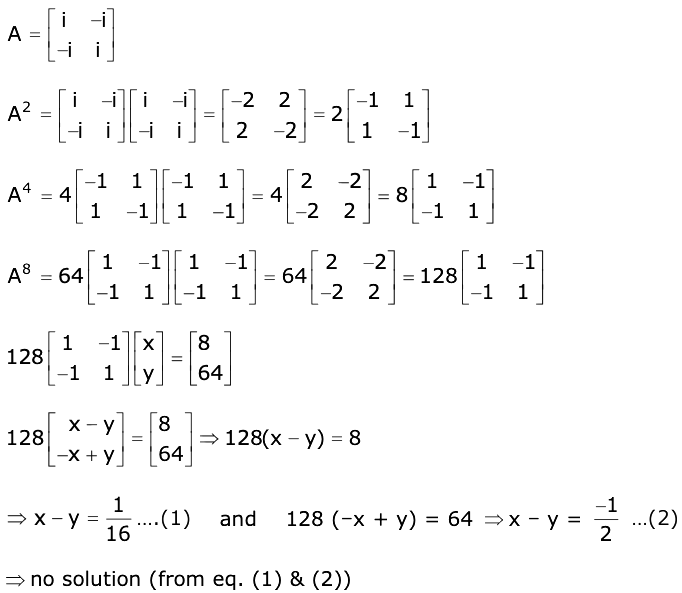Question 16: If for x ∈ (0, π / 2), log10sin x + log10cos x = –1 and log10(sin x+cos x) = 1 / 2 (log10 n – 1), n>0, then the value of n is equal to:

1. a. 16
2. b. 20
3. c. 12
4. d. 9

Solution:

log10sin x + log10cos x = –1

sinx * cosx = 1 / 10 --- (1)

log10 (sinx + cosx) = (1 / 2) (log10 n - 1)

(sinx + cosx) = (n / 10)½

sin2 x + cos2 x + 2 sinx cosx = n / 10 (squaring)

1 + 2 (1 / 10) = n / 10 [using equation (1)]

n / 10 = 12 / 10

n = 12

Question 17: The locus of the midpoints of the chord of the circle, x2 + y2 = 25 which is tangent to the hyperbola, x2 / 9 - y2 / 16 = 1 is :

1. a. (x2 + y2)2 - 16x2 + 9y2 = 0
2. b. (x2 + y2)2 - 9x2 + 144y2 = 0
3. c. (x2 + y2)2 - 9x2 - 16y2 = 0
4. d. (x2 + y2)2 - 9x2 + 16y2 = 0

Solution:

The tangent of hyperbola y = mx ± √(9m2 - 16) …(i) which is a chord of circle with mid-point (h, k).

So, the equation of chord T = S1

hx + ky = h2 + k2

y = - (hx / k) + (h2 + k2) / k ---- (ii)

By (i) and (ii)

m = - h / k and √9m2 - 16 = [h2 + k2] / k

9 (h2 / k2) - 16 = (h2 + k2)2 / k2

The locus 9x2 – 16y2 = (x2 + y2)2.

Question 18: Let [x] denote the greatest integer less than or equal to x. If for n ∈ N, (1 - x + x3)n = ∑j=03n ajxj, then ∑j=03n/2 a2j + 4 ∑j=0(3n-1)/2 a2j+1 =

1. a. 1
2. b. n
3. c. 2n–1
4. d. 2

Solution:

(1 - x + x3)n = ∑j=03n ajxj

(1–x + x3)n = a0 + a1x + a2x2 + ….. + a3n x3n

Put x = 1

1 = a0 + a1 + a2 + a3 + a4 + …… +a3n …(1)

Put x = –1

1 = a0 – a1 + a2 – a3 + a4 …… (–1)3na3n …(2)

a0 + a2 + a4 + a6 + …….. = 1

Sub (1) – (2)

⇒ a1 + a3 + a5 + a7 + …….. = 0

Now ∑j=03n/2 a2j + 4 ∑j=0(3n-1)/2 a2j+1 =

= (a0 + a2 + a4 + …..) + 4(a1 + a3+ ….)

= 1 + 4 × 0

= 1

Question 19: Let P be a plane lx + my + nz = 0 containing the line, [1 - x] / 1 = [y + 4] / 2 = [z + 2] / 3. If plane P divides the line segment AB joining points A (–3, –6, 1) and B (2, 4, –3) in ratio k : 1 then the value of k is equal to :

1. a. 1.5
2. b. 2
3. c. 4
4. d. 3

Solution:Line lies on plane

– l + 2m + 3n = 0 ...(1)

Point on line (1,– 4, – 2) lies on plane

l – 4m – 2n = 0 …(2)

From (1) & (2)

–2m + n = 0

2m = n

l = 3n + 2m

l = 4n

l : m : n :: 4n : (n / 2) : n

l : m : n :: 8n : (n) : 2n

l : m : n :: 8 : 1 : 2

Now equation of plane is 8x + y + 2z = 0

R divide AB is ratio k : 1

R : [(- 3 + 2k) / (k + 1), (- 6 + 4k) / (k + 1), (1 - 3k) / (k + 1)] lies on the plane

8 [(- 3 + 2k) / (k + 1) + (- 6 + 4k) / (k + 1) + 2 (1 - 3k) / (k + 1)] = 0

–24 + 16 k – 6+ 4k + 2 – 6k = 0

–28 + 14k = 0

k = 2

Question 20: The number of elements in the set x ∈ R : (|x| - 3) |x + 4| = 6) =

1. a. 2
2. b. 1
3. c. 3
4. d. 4

Solution:

Case - 1: x ≤ – 4

(–x – 3)(–x – 4) = 6

(x + 3) (x + 4) = 6

x2 + 7x + 6 = 0

x = –1 or – 6

But x ≤ – 4

x = – 6

Case - 2: x ∈ (– 4, 0)

(–x – 3)(x + 4) = 6

–x2 – 7x – 12 – 6 = 0

x2 + 7x + 18 = 0

D < 0 No solution

Case - 3: x ≥ 0

(x – 3)(x + 4) = 6

x2 + x – 12 – 6 = 0

x2 + x – 18 = 0

x = (- 1 ± √73) / 2

Only x = (√73 - 1) / 2

Hence, 2 elements only

Section - B

Question 21: Let f: (0, 2) → R be defined as f (x) = log2 [1 + tan (πx / 4)]. Then, limn→∞ (2 / n) [f (1 / n) + f (2 / n) ……. f (1)] is equal to ______

Solution: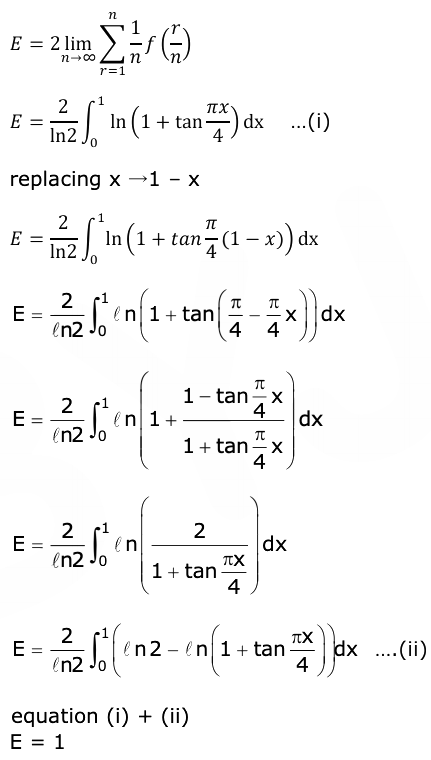Question 22: The total number of 3 × 3 matrices A having entries from the set {0, 1, 2, 3} such that the sum of all the diagonal entries of AAT is 9, is equal to ____

Solution:

$$AA^T=\begin{bmatrix} x &y &z \\ a&b &c \\ d &e &f \end{bmatrix}\begin{bmatrix} x&a &d \\ y&b &e \\ z&c &f \end{bmatrix}\\ =\begin{bmatrix} x^2+y^2+z^2 &ax+by+cz &dx+ey+fz \\ ax+by+cz& a^2+b^2+c^2 &ad+be+cf \\ dx+ey+fz &ad+be+cf & d^2+e^2+f^2 \end{bmatrix}$$

Tr (AAT) = x2 + y2 + z2 + a2 + b2 + c2 + d2 + e2 + f2 = 9

all→1 =1

one 3, rest = 0 (9! / 8!) = 9

two 2 , one 1 & rest 0 (9!) / (2! 6!) = 63 × 4 = 252

one 2 , five 1, rest 0 (9!) / (5! 3!) = 63 × 8 = 504

Total = 766

Question 23: Let f:R→R be a continuous function such that f (x) + f (x + 1) = 2, for all x ∈ R. If I1 = ∫08 f (x) dx and I2 = ∫-13 f (x) dx, then the value of I1 + 2I2 is equal to ____

Solution:

f (x) + f (x + 1) = 2 …..(i)

x→(x + 1)

f(x + 1) + f(x + 2) = 2 …..(ii)

By (i) & (ii)

f(x) – f(x + 2) = 0

f(x + 2) = f(x)

f(x) is periodic with T = 2

I1 = ∫02*4 f (x) dx = 4 ∫02 f (x) dx

I2 = ∫-13 f (x) dx = ∫04 f (x + 1) dx = ∫04 [2 - f (x)] dx

I2 = 8 - 2 ∫02 f (x) dx

I1 + 2I2 = 16

Question 24: Consider an arithmetic series and a geometric series having four initial terms from the set {11, 8, 21, 16, 26, 32, 4}. If the last terms of these series are the maximum possible four-digit numbers, then the number of common terms in these two series is equal to ____

Solution:

By observation

A.P : 11, 16, 21, 26 …….

G.P : 4, 8, 16, 32 …….

So common terms are 16, 256, 4096.

Question 25: If the normal to the curve y (x) = ∫0x (2t2 - 15t + 10) dt at a point (a, b) is parallel to the line x + 3y = – 5, a>1, then the value of |a + 6b| is equal to ____

Solution:

y' (x) = (2x2 – 15x + 10)

At point (a, b) normal is

3 = (2a2 – 15a + 10)

2a2 – 15a + 7 = 0

2a2 – 14a – a + 7 = 0

2a (a – 7) – 1 (a – 7) = 0

a = 1 / 2 or 7,

Given a > 1, a = 7

Also P lies on curve,

b = ∫0a (2t2 - 15t + 10) dt

b = ∫07 (2t2 - 15t + 10) dt

6b = – 413

|a + 6b| = 406

Question 26: If limx→0 [aex - b cosx + ce-x] / [x sinx] = 2, then a + b + c is equal to ______

Solution: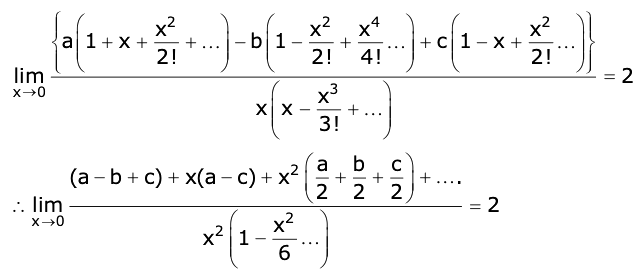a – b + c = 0 & a – c = 0 & (a / 2) + (b / 2) + (c / 2) = 2

a + b + c = 4

Question 27: Let ABCD be a square of the side of unit length. Let a circle C1 centred at A with a unit radius is drawn. Another circle C2 which touches C1 and the lines AD and AB is tangent to it, is also drawn. Let a tangent line from point C to the circle C2 meet the side AB at E. If the length of EB is ɑ + √3β, where ɑ, β are integers, then ɑ + β is equal to ___

Solution: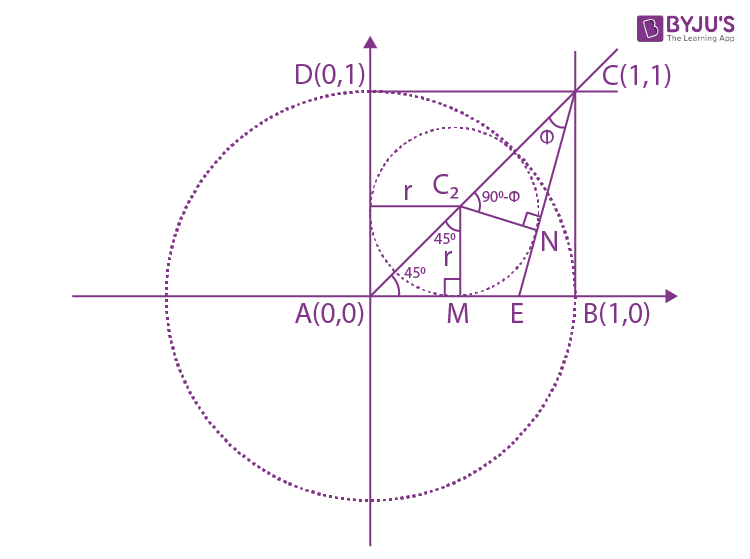(i) √2r + r = 1

r = 1 / (√2 + 1)

r = √2 - 1

(ii) CC2 = 2√2 - 2 = 2 (√2 - 1)

From △CC2N = sin ɸ = (√2 - 1) / [2 (√2 - 1)]

ɸ = 30o

(iii) In △ACE are sine law

AE / sin ɸ = AC / sin 105o

AE = (1 / 2) * (√2 / (√3 + 1)) * 2√2

AE = 2 / (√3 + 1) = √3 - 1

EB = 1 - (√3 - 1)

ɑ = 2, β = - 1

ɑ + β = 1

Question 28: Let z and w be two complex numbers such that

$$w=z\bar{z}-2z+2$$
, |(z + i) / (z - 3i)| = 1 and Re (w) has a minimum value. Then, the minimum value of n ∈ N, for which wn is real, is equal to ____

Solution:

Let z = x + iy

|z + i| = |z–3i|

y = 1

Now w = x2 + y2 – 2x – 2iy + 2

w = x2 + 1 – 2x –2i + 2

Re(w) = x2 – 2x + 3

Re(w) = (x–1)2 + 2

Re(w)min at x = 1

z = 1 + i

Now w = 1 + 1 –2 – 2i + 2

w = 2(1–i) = 2√2 ei(-π/4)

wn = 2√2 ei(-π/4)

If wn is real, n = 4

Question 29: Let

$$P=\begin{bmatrix} -30 &20 &56 \\ 90&140 &112 \\ 120&60 &14 \end{bmatrix}$$
and
$$A=\begin{bmatrix} 2 &7 &\omega ^2 \\ -1&-\omega &1 \\ 0&-\omega &-\omega+1 \end{bmatrix}$$
where 𝛚 = (- 1 + i √3) / 2, and I3 be the identity matrix of order 3. If the determinant of the matrix (P-1AP- I3)2 is ɑ𝛚2, then the value of ɑ is equal to _____

Solution:

|P-1AP - I|2

= |(P-1AP - I) (P-1AP - I)|

= |P-1APP-1 AP - 2P-1AP + I|

= |P-1A2P - 2P-1AP + P-1IP|

= |P-1(A2 - 2A + I) P|

= |P-1(A - I)2 P|

= |P-1| |A - I|2 |P|

= |A - I|2

=

$$=\begin{bmatrix} 1 &7 &\omega ^2 \\ -1&-\omega-1 &1 \\ 0&-\omega &-\omega \end{bmatrix}^2$$

= (1 (𝛚 (𝛚 + 1) + 𝛚) - 7𝛚 + 𝛚2 . 𝛚)2

= (𝛚2 + 2𝛚 - 7𝛚 + 1)2

= (𝛚2 - 5𝛚 + 1)2

= (- 6𝛚)2

= 36𝛚2 ⇒ ɑ = 36

Question 30: Let the curve y=y(x) be the solution of the differential equation, dy / dx = 2 (x + 1). If the numerical value of area bounded by the curve y = y(x) and the x-axis is 4√8 / 3, then the value of y(1) is equal to ___

Solution:

y = x2 + 2x + c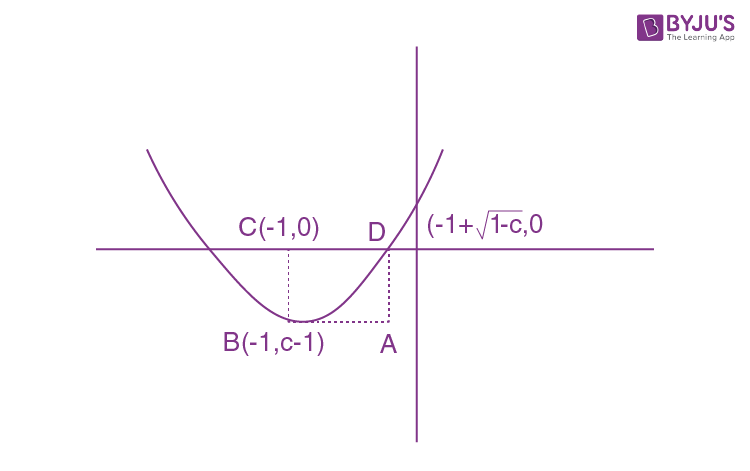Area of rectangle ABCD = |(c - 1)√(1 - c)|

Area of parabola and x-axis = 2 (2 / 3 [(1 - c)3/2]) = 4√8 / 3

1 – c = 2

c = –1

Equation of f (x) = x2 + 2x – 1

f (1) = 1 + 2 – 1 = 2

### JEE Main 2021 Maths Paper March 16 Shift 1